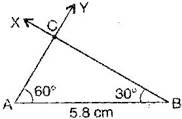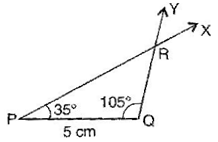### Practical Geometry - Solutions 4

CBSE Class –VII Mathematics
NCERT Solutions
Chapter 10 Practical Geometry (Ex. 10.4)

Question 1. Construct $\mathrm{\Delta }$ABC, given $m\mathrm{\angle }$A = ${60}^{\circ },$$m\mathrm{\angle }$B = ${30}^{\circ }$ and AB = 5.8 cm.
Answer: To construct$\mathrm{\Delta }$ABC where $m\mathrm{\angle }$A = ${60}^{\circ },$$m\mathrm{\angle }$B = ${30}^{\circ }$ and AB = 5.8 cm.Steps of construction:
(a) Draw a line segment AB = 5.8 cm.
(b) At point A, draw an angle $\mathrm{\angle }$YAB = ${60}^{\circ }$ with the help of compass.
(c) At point B, draw $\mathrm{\angle }$XBA = ${30}^{\circ }$ with the help of compass.
(d) AY and BX intersect at the point C.
It is the required triangle ABC.
Question 2. Construct $\mathrm{\Delta }$PQR if PQ = 5 cm, $m\mathrm{\angle }$PQR = ${105}^{\circ }$ and $m\mathrm{\angle }$QRP = ${40}^{\circ }.$
Answer: Given$m\mathrm{\angle }$PQR = ${105}^{\circ }$ and $m\mathrm{\angle }$QRP = ${40}^{\circ }$
We know that sum of angles of a triangle is ${180}^{\circ }.$
$\therefore$ $m\mathrm{\angle }$PQR + $m\mathrm{\angle }$QRP + $m\mathrm{\angle }$QPR = ${180}^{\circ }$
$⇒$ ${105}^{\circ }+{40}^{\circ }+m\mathrm{\angle }$QPR = ${180}^{\circ }$
$⇒$ ${145}^{\circ }$ + $m\mathrm{\angle }$QPR = ${180}^{\circ }$
$⇒$ $m\mathrm{\angle }$QPR = ${180}^{\circ }$ – ${145}^{\circ }$
$⇒$ $m\mathrm{\angle }$QPR = ${35}^{\circ }$To construct$\mathrm{\Delta }$PQR where $m\mathrm{\angle }$P = ${35}^{\circ }$$m\mathrm{\angle }$Q = ${105}^{\circ }$ and PQ = 5 cm.
Steps of construction:
(a) Draw a line segment PQ = 5 cm.
(b) At point P, draw $\mathrm{\angle }$XPQ = ${35}^{\circ }$ with the help of protractor.
(c) At point Q, draw $\mathrm{\angle }$YQP = ${105}^{\circ }$ with the help of protractor.
(d) XP and YQ intersect at point R.
It is the required triangle PQR.
Question 3. Examine whether you can construct $\mathrm{\Delta }$DEF such that EF = 7.2 cm, $m\mathrm{\angle }$E = ${110}^{\circ }$ and $m\mathrm{\angle }$F = ${80}^{\circ }.$ Justify your answer.
Answer: Triangel DEF cannot be constructed since Angle E + Angle F = 110o + 80o = 190o
and the sum of all the angles of triangle is 180o.